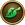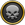8,432 Pages

This Is A Stub Please Help Us By Expanding It. {{CreatureInfobox | image = | cretype = Boss | rank = 5 | heal = 1200 | crecla = Raven | school = Life | damperpip = 85 | damsch = Death | minion = | minion2 = | world = Grizzleheim | location = Frostholm | descrip = Also see [[This Is A Stub Please Help Us By Expanding It. {{CreatureInfobox | image = | cretype = Boss | rank = 7 | heal = 4200 | crecla = Raven | school = Life | damperpip = 85 | damsch = Death | minion = | minion2 = | world = Grizzleheim | location = Ravenscar | descrip = Also see [[Mor, [[This Is A Stub Please Help Us By Expanding It.

Description

Also see [[Mor, Mord Runechanter (Ravenscar Boss) and Mord Runechanter (NPC)

You will fight Mord Runechanter THREE TIMES (?) as you progress through Grizzleheim. The RANK 5 version is a boss in Frostholm (?). Later, you fight the FIRST RANK 7 version in Ravenscar before you fight him THIS TIME with the rest of The Coven in Ravenscar. | spell1 = Life Trap | spell2 = Lifeblade | spell3 = Leprechaun | spell4 = Sprite | spell5 = Spirit Armor | spell6 = Seraph | spell7 = Centaur | spell8 = Storm Shark | spell9 = Cyclops | spell10 = Weakness | spell11 = Death Shield | spell12 = | spell13 = | spell14 = | gold = | hat1 = | hat2 = | hat3 = | hat4 = | hat5 = | hat6 = | hat7 = | hat8 = | hat9 = | hat10 = | robe1 = | robe2 = | robe3 = | robe4 = | robe5 = | robe6 = | robe7 = | robe8 = | robe9 = | robe10 = | boots1 = | boots2 = | boots3 = | boots4 = | boots5 = | boots6 = | boots7 = | boots8 = | boots9 = | boots10 = | wand1 = | wand2 = | wand3 = | wand4 = | wand5 = | wand6 = | wand7 = | wand8 = | wand9 = | wand10= | athame1 = | athame2 = | athame3 = | athame4 = | athame5 = | athame6 = | athame7 = | athame8 = | athame9 = | athame10 = | amulet1 = | amulet2 = | amulet3 = | amulet4 = | amulet5 = | amulet6 = | amulet7 = | amulet8 = | amulet9 = | amulet10 = | ring1 = | ring2 = | ring3 = | ring4 = | ring5 = | ring6 = | ring7 = | ring8 = | ring9 = | ring10 = | deck1 = | deck2 = | deck3 = | deck5 = | deck6 = | deck7 = | deck8 = | deck9 = | deck10 = | pet1 = | pet2 = | pet3 = | pet4 = | pet5 = | pet6 = | pet7 = | pet8 = | pet9 = | pet10 = | house1 = | house2 = | house3 = | house4 = | house5 = | house6 = | house7 = | house8 = | house9 = | house10 = | trecar1 = | trecar2 = | trecar3 = | trecar4 = | trecar5 = | trecar6 = | trecar7 = | trecar8 = | trecar9 = | trecar10 = | reag1 = | reag2 = | reag3 = | reag4 = | reag5 = | reag6 = | reag7 = | reag8 = | reag9 = | reag10 = | snack1 = | snack2 = | snack3 = | snack4 = | snack5 = | snack6 = | snack7 = | snack8 = | snack9 = | snack10 = | mount1 = | mount2 = | quest1 = | quest2 = | quest3 = }} (Ravenscar Boss)]] and Mord Runechanter (NPC)

You will fight Mord Runechanter THREE TIMES (?) as you progress through Grizzleheim. The RANK 5 version is a boss in Frostholm (?). Later, you fight the FIRST RANK 7 version in Ravenscar before you fight him THIS TIME with the rest of The Coven in Ravenscar.

Mord Runechanter (Frostholm boss) at a Glance:
 Rank: 7 Boss Health: 4200 Creature Class: Raven School:Boost: 50% boost toResist: 80% resist toPhysical Attack: Deals 85damage per pip. Location: Grizzleheim- Ravenscar

Spells Known

Items Dropped

Gold:
Hats:
Robes:
Boots:
Wands:
Athames:
Amulets:
Rings:
Decks:
Pets:
House:
Treasure Cards:
Reagents:
Snacks:
Mounts:

Quest Appearances

Hints, Guides and Discussions should be placed in the Discussion tab.

Documentation on how to edit this page can be found at Template:CreatureInfobox/doc

(Ravenscar Boss)]] and Mord Runechanter (NPC) He You will fight Mord Runechanter THREE TIMES (?) as you progress through Grizzleheim. The RANK 5 version is a boss in Frostholm and imposer of Ivar Anderson (?). Later, you fight the FIRST RANK 7 version in Ravenscar before you fight him THIS TIME with the rest of The Coven in Ravenscar. | spell1 = Life Trap | spell2 = Lifeblade | spell3 = Leprechaun | spell4 = Sprite | spell5 = Spirit Armor | spell6 = Seraph | spell7 = Centaur | spell8 = Storm Shark | spell9 = Cyclops | spell10 = Weakness | spell11 = Death Shield | spell12 = | spell13 = | spell14 = | gold = | hat1 = | hat2 = | hat3 = | hat4 = | hat5 = | hat6 = | hat7 = | hat8 = | hat9 = | hat10 = | robe1 = | robe2 = | robe3 = | robe4 = | robe5 = | robe6 = | robe7 = | robe8 = | robe9 = | robe10 = | boots1 = | boots2 = | boots3 = | boots4 = | boots5 = | boots6 = | boots7 = | boots8 = | boots9 = | boots10 = | wand1 = | wand2 = | wand3 = | wand4 = | wand5 = | wand6 = | wand7 = | wand8 = | wand9 = | wand10= | athame1 = | athame2 = | athame3 = | athame4 = | athame5 = | athame6 = | athame7 = | athame8 = | athame9 = | athame10 = | amulet1 = | amulet2 = | amulet3 = | amulet4 = | amulet5 = | amulet6 = | amulet7 = | amulet8 = | amulet9 = | amulet10 = | ring1 = | ring2 = | ring3 = | ring4 = | ring5 = | ring6 = | ring7 = | ring8 = | ring9 = | ring10 = | deck1 = | deck2 = | deck3 = | deck5 = | deck6 = | deck7 = | deck8 = | deck9 = | deck10 = | pet1 = | pet2 = | pet3 = | pet4 = | pet5 = | pet6 = | pet7 = | pet8 = | pet9 = | pet10 = | house1 = | house2 = | house3 = | house4 = | house5 = | house6 = | house7 = | house8 = | house9 = | house10 = | trecar1 = | trecar2 = | trecar3 = | trecar4 = | trecar5 = | trecar6 = | trecar7 = | trecar8 = | trecar9 = | trecar10 = | reag1 = | reag2 = | reag3 = | reag4 = | reag5 = | reag6 = | reag7 = | reag8 = | reag9 = | reag10 = | snack1 = | snack2 = | snack3 = | snack4 = | snack5 = | snack6 = | snack7 = | snack8 = | snack9 = | snack10 = | mount1 = | mount2 = | quest1 = | quest2 = | quest3 = }}

Community content is available under CC-BY-SA unless otherwise noted.